Next: C. Proofs Up: B. Algorithms Previous: B.2 Kernel PCA speed-up

# B.3 Quality measure for a potential field

In this section a method is introduced that determines the quality of the match between a potential field and a data distribution {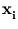}. The overlap is computed between the data distribution and a region of same volume enclosed by an iso-potential curve (figure B.1). The method relies on the data points being uniformly distributed over a closed region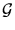with volume A (as it is the case for the ring-line-square and vortex distributions).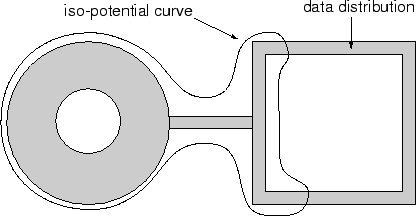Let Bc be the volume of the closed region defined by {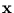| p()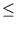c}, which is the set of points surrounded by an iso-potential curve with value c. The volume Bc was calculated using Monte-Carlo integration.

The computation of the quality measure has two steps. First, choose c, such that Bc = A. Second, count the number of data pointsfulfilling p()c. The quality measure is the percentage of this number on the total number of data points.Next: C. Proofs Up: B. Algorithms Previous: B.2 Kernel PCA speed-up
Heiko Hoffmann
2005-03-22